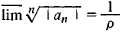Also found in: Wikipedia.

(mathematics)
The theorem that the radius of convergence of a Taylor series in the complex variable z is the reciprocal of the limit superior, as n approaches infinity, of the n th root of the absolute value of the coefficient of zn.
McGraw-Hill Dictionary of Scientific & Technical Terms, 6E, Copyright © 2003 by The McGraw-Hill Companies, Inc.
The following article is from The Great Soviet Encyclopedia (1979). It might be outdated or ideologically biased.

a theorem of the theory of analytic functions that permits the evaluation of the convergence of the power series

a0 + a1(z − z0) + … an(z − z0)n + …

where a0, a1, …, an are fixed complex numbers and z is a complex variable. The Cauchy-Hadamard theorem states that if the upper limitthen when ρ = ∞ the series converges absolutely in the entire plane. When ρ = 0 the series converges only at the point z = z0 and diverges when z ≠ z0. Finally, for the case 0 < ρ < ∞, the series converges absolutely in the circle ǀ z − Z0ǀ< ρ and diverges outside it. The theorem was established by A. Cauchy (1821), and a second proof of it was given by J. Hadamard (1888), who indicated its important applications.